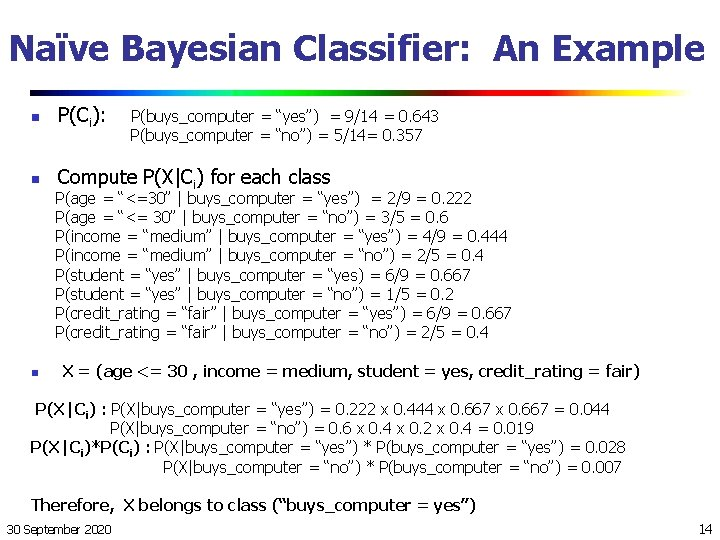# Probabilistic Reasoning and Bayesian Belief Networks 1 Bayes

• Slides: 15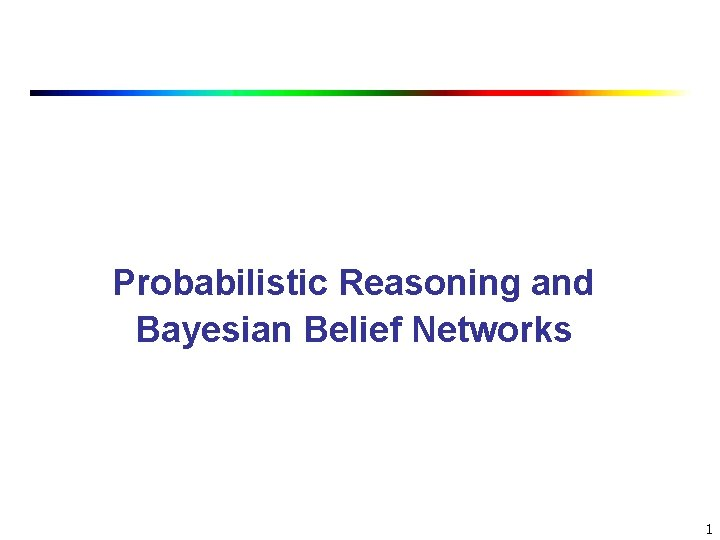Probabilistic Reasoning and Bayesian Belief Networks 1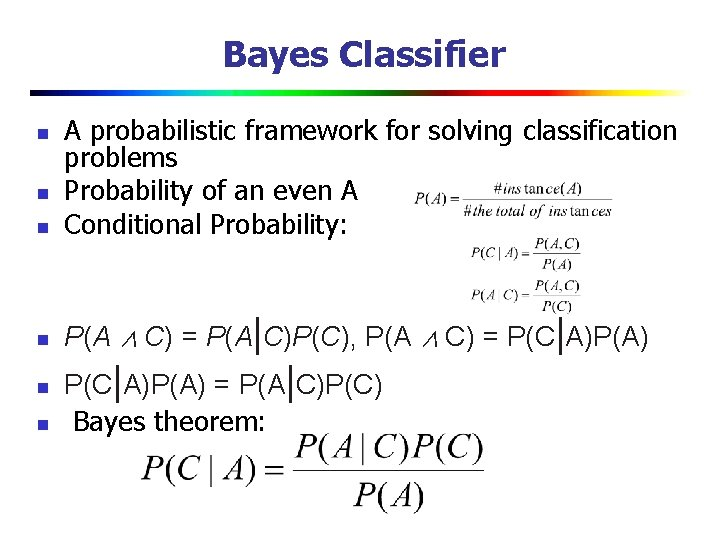Bayes Classifier n A probabilistic framework for solving classification problems Probability of an even A Conditional Probability: n P(A ∧ C) = P(A|C)P(C), P(A ∧ C) = P(C|A)P(A) n n P(C|A)P(A) = P(A|C)P(C) Bayes theorem: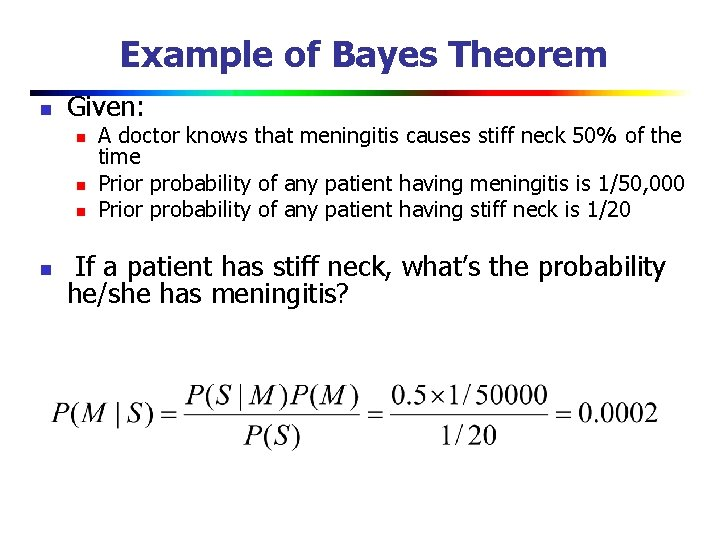Example of Bayes Theorem n Given: n n A doctor knows that meningitis causes stiff neck 50% of the time Prior probability of any patient having meningitis is 1/50, 000 Prior probability of any patient having stiff neck is 1/20 If a patient has stiff neck, what’s the probability he/she has meningitis?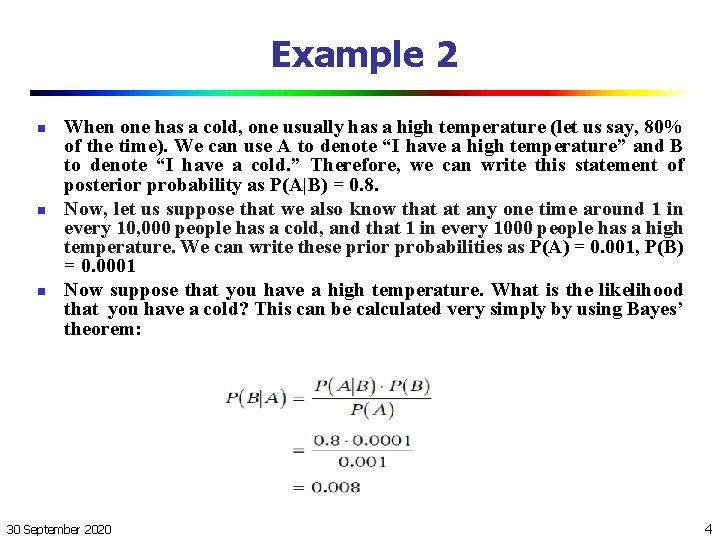Example 2 n n n When one has a cold, one usually has a high temperature (let us say, 80% of the time). We can use A to denote “I have a high temperature” and B to denote “I have a cold. ” Therefore, we can write this statement of posterior probability as P(A|B) = 0. 8. Now, let us suppose that we also know that at any one time around 1 in every 10, 000 people has a cold, and that 1 in every 1000 people has a high temperature. We can write these prior probabilities as P(A) = 0. 001, P(B) = 0. 0001 Now suppose that you have a high temperature. What is the likelihood that you have a cold? This can be calculated very simply by using Bayes’ theorem: 30 September 2020 4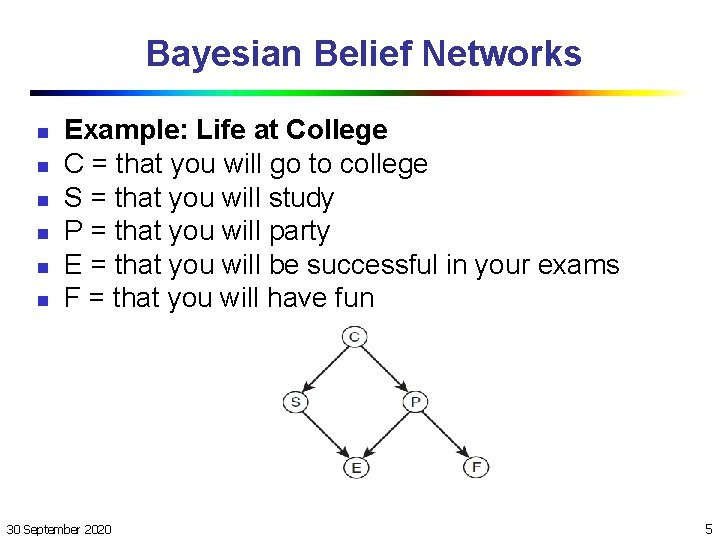Bayesian Belief Networks n n n Example: Life at College C = that you will go to college S = that you will study P = that you will party E = that you will be successful in your exams F = that you will have fun 30 September 2020 5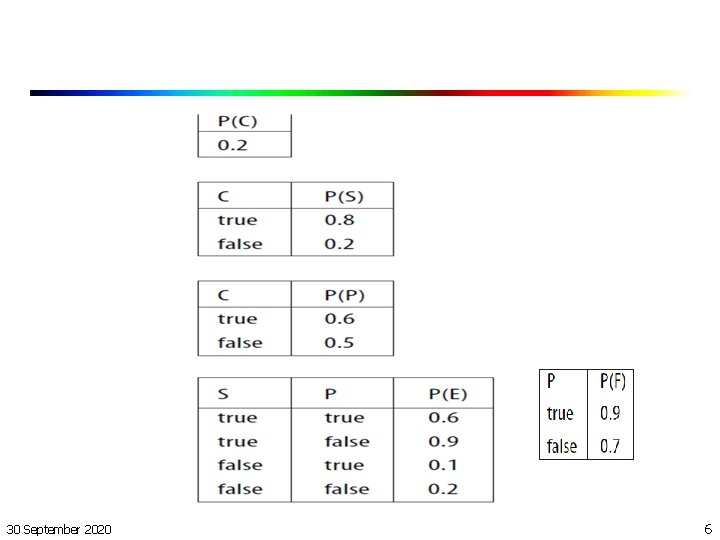30 September 2020 6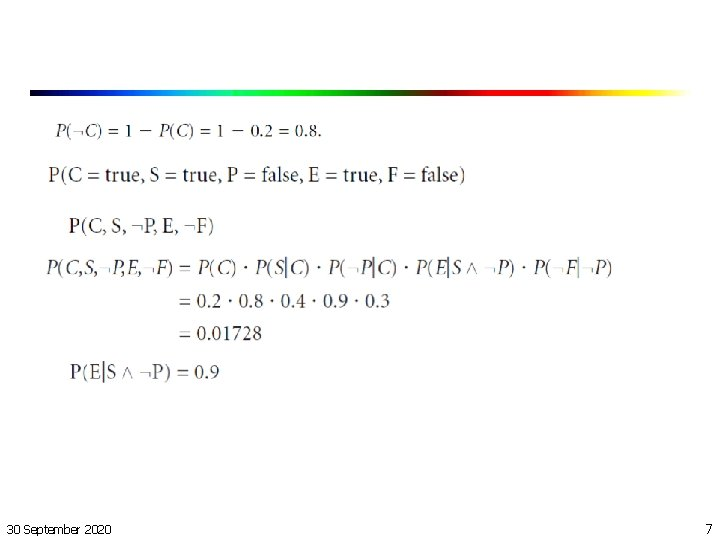30 September 2020 7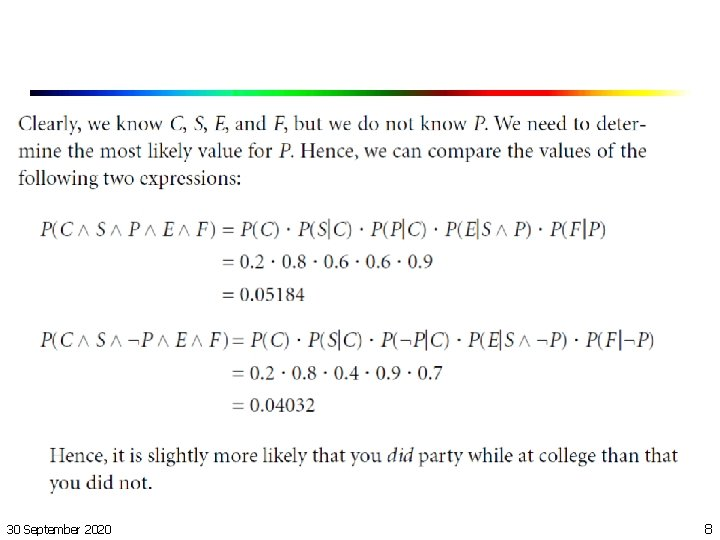30 September 2020 8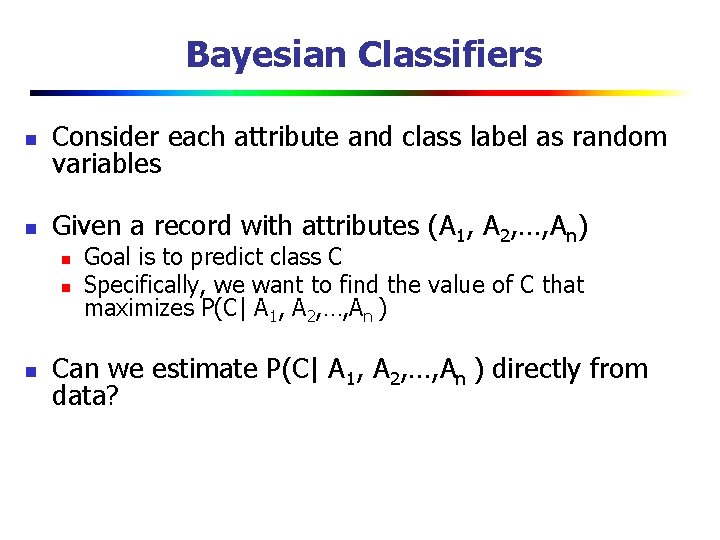Bayesian Classifiers n Consider each attribute and class label as random variables n Given a record with attributes (A 1, A 2, …, An) n n n Goal is to predict class C Specifically, we want to find the value of C that maximizes P(C| A 1, A 2, …, An ) Can we estimate P(C| A 1, A 2, …, An ) directly from data?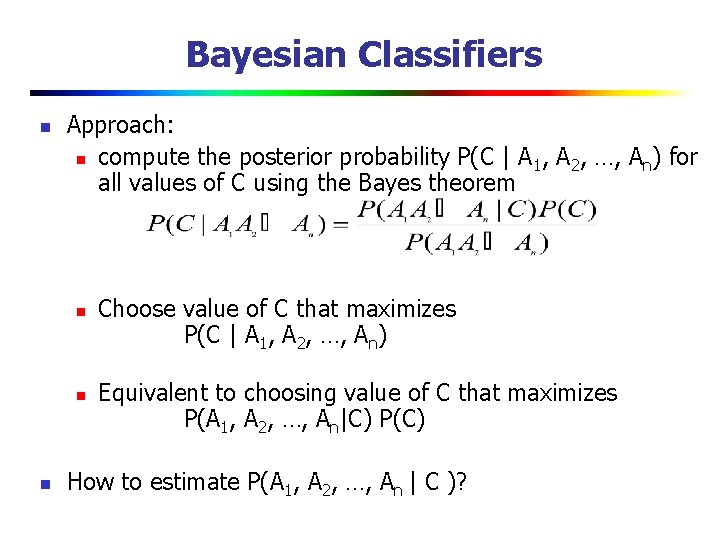Bayesian Classifiers n Approach: n compute the posterior probability P(C | A 1, A 2, …, An) for all values of C using the Bayes theorem n n n Choose value of C that maximizes P(C | A 1, A 2, …, An) Equivalent to choosing value of C that maximizes P(A 1, A 2, …, An|C) P(C) How to estimate P(A 1, A 2, …, An | C )?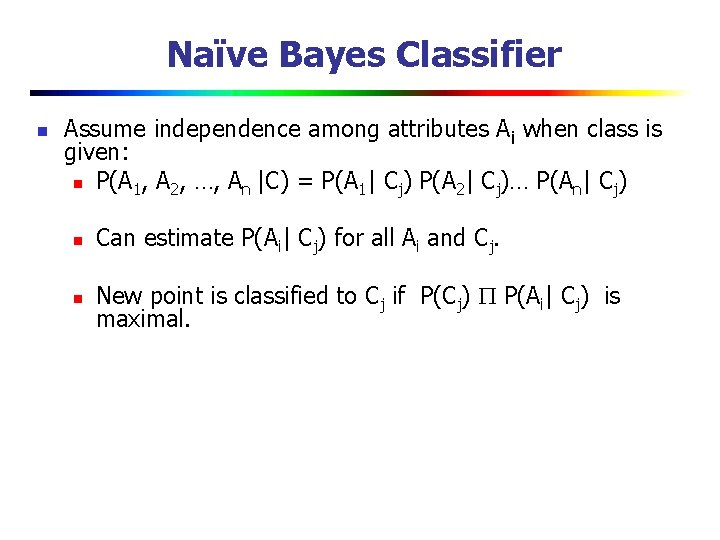Naïve Bayes Classifier n Assume independence among attributes Ai when class is given: n P(A 1, A 2, …, An |C) = P(A 1| Cj) P(A 2| Cj)… P(An| Cj) n Can estimate P(Ai| Cj) for all Ai and Cj. n New point is classified to Cj if P(Cj) P(Ai| Cj) is maximal.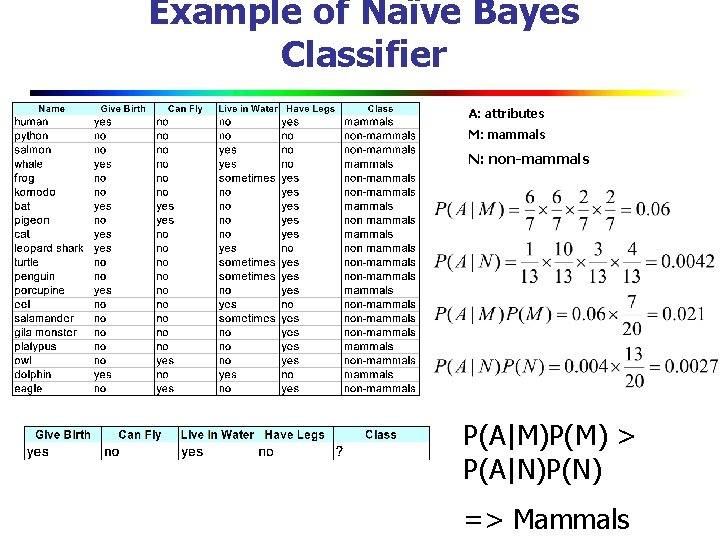Example of Naïve Bayes Classifier A: attributes M: mammals N: non-mammals P(A|M)P(M) > P(A|N)P(N) => MammalsNaïve Bayesian Classifier: Training Dataset Class: C 1: buys_computer = ‘yes’ C 2: buys_computer = ‘no’ Data sample X = (age <=30, Income = medium, Student = yes Credit_rating = Fair) 30 September 2020 13